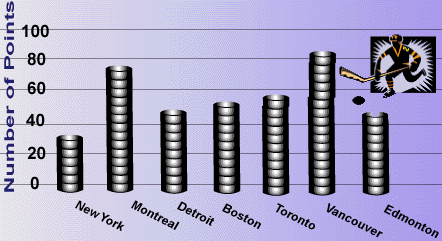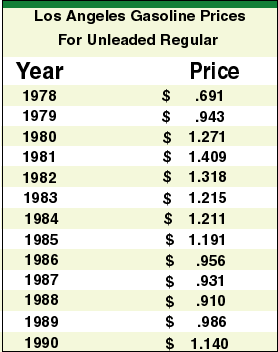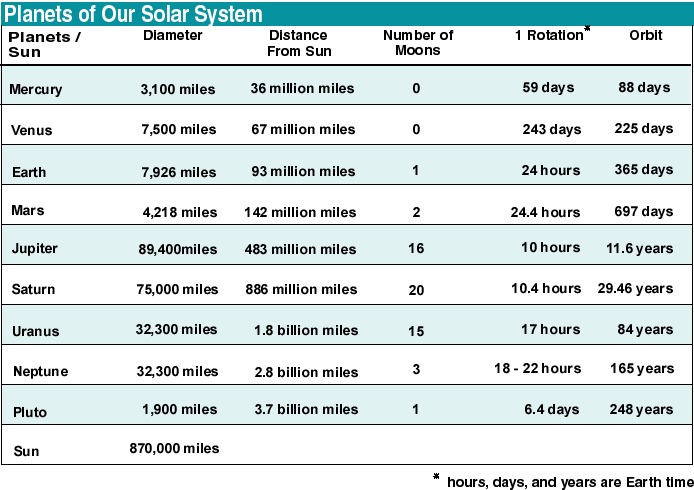<This activity was developed by a student or students at Mainland High School which is located in Daytona Beach, FL.   It is still a "work in progress" with editing and improvements yet to come.

Bar Graphs
help to make comparisons among several items in a given category. To make a bar graph, follow these steps below.Draw two perpendicular axes on grid paper. Label each axes to identify the variables.Choose a scale that will permit the full range of values to be graphed. Mark one axis with equal intervals.Mark the other axis with equal intervals. These intervals do not have to match those used on the other axis.Using the data values, carefully find the height of each bar for each item. Draw and shade each bar. Be sure to leave space between your bars.

4. Study the bar graph shown below.a. What is being compared in this bar graph?
b. What conclusions can you make from the data show by the bar graph?

5. Refer to your data from  your class questionnaire survey on page 24 of your Portfolio Builder. Use the data from one question to make a bar graph.

Line graphs are used to show the behavior of a variable. To make a line graph, follow the steps below.Draw two axes on grid paper as described before. The horizontal axis usually shows units of time, and the vertical axis represents the quantity being studied.Graph the points for the data and then connect the points from left to right with line segments.6. With your partner, study and discuss the following data.

a. Make a line graph of the data.
b. What is the year with the highest annual gasoline?
c. What is the greatest difference when comparing consecutive years? Why might it be different each month?
d. How can you use the graph to find the median? the mode? the mean? the range?
e. What is the overall pattern?Using the information from the chart, make a scatter plot using the data from the two different columns in the solar system chart. Here are some ideas for scatter plots.planet diameter and distance from the sunplanet diameter and number of moonsplanet diameter and time for one  rotationplanet diameter and time for one orbitdistance from the sun and number of moonsdistance from the sun and time for one orbitdistance from the sun and time for one rotationnumber of moons and time for one orbitnumber of moons and time for one rotationtime for one rotation and time for one orbit

a. Are there any patterns suggested by your scatter plot?
b. Compare and discuss your results with other students. What are the most interesting relationships found? Why?

8. The table shows the winning times (in minutes) for the men's Olympic marathon from 1896 to 1988. The times are rounded to the nearest minute.

a. Construct an appropriate graph for this data.
b. Find the three dates when there is no data. Why are these dates missing?
c. When did the greatest improvement in the winning time occur? Why do you think this is true?
d. What do you predict for the winning time in 1996? 2000? 2020?

9. Using newspapers, collect one example of each kind of graph you have learned about. Mount the graph on a sheet of paper. Note the type of graph and it's source. Write a brief explanation of any conclusions you found from the graph.

 MathSummer Activity Summary Copyright 1997-1999: html adaptation Career Connection to Teaching with Technology